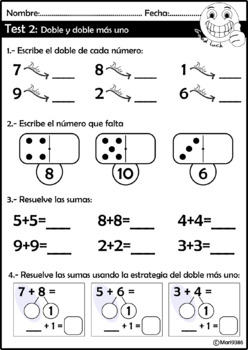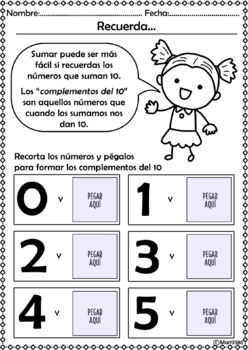Subject
Resource Type
File Type

Word Document File

(136 MB|144 pages)
Product Rating
4.0
(2 Ratings)
Standards
• Product Description
• StandardsNEW

In this product you will found more than 140 pages of games, cards, worksheets and everything you need to teach adding to 20.

This pack comes with these concepts/standards:

. * Counting on using fingers, dice and domino.

. * Numbers lines with sums to 19 (counting forward)

. * Making 10 (tens complement)

. * Word problems with sums to 20

. * Writing addition number sentences

. * Doubles

. * Doubles plus one

. * Fact fluency

. * Making a ten to add

You will find worksheets, cut and paste activities and games that will make your students stay motivated.

Please click the PREVIEW button above to see a free sample of the activities that are included in this product.

See this product in ENGLISH version

Please visit my store for more math activities

Determine the unknown whole number in an addition or subtraction equation relating three whole numbers. For example, determine the unknown number that makes the equation true in each of the equations 8 + ? = 11, 5 = ▯ - 3, 6 + 6 = ▯.
Understand the meaning of the equal sign, and determine if equations involving addition and subtraction are true or false. For example, which of the following equations are true and which are false? 6 = 6, 7 = 8 - 1, 5 + 2 = 2 + 5, 4 + 1 = 5 + 2.
Add and subtract within 20, demonstrating fluency for addition and subtraction within 10. Use strategies such as counting on; making ten (e.g., 8 + 6 = 8 + 2 + 4 = 10 + 4 = 14); decomposing a number leading to a ten (e.g., 13 - 4 = 13 - 3 - 1 = 10 - 1 = 9); using the relationship between addition and subtraction (e.g., knowing that 8 + 4 = 12, one knows 12 - 8 = 4); and creating equivalent but easier or known sums (e.g., adding 6 + 7 by creating the known equivalent 6 + 6 + 1 = 12 + 1 = 13).
Apply properties of operations as strategies to add and subtract. If 8 + 3 = 11 is known, then 3 + 8 = 11 is also known. (Commutative property of addition.) To add 2 + 6 + 4, the second two numbers can be added to make a ten, so 2 + 6 + 4 = 2 + 10 = 12. (Associative property of addition.)
Solve word problems that call for addition of three whole numbers whose sum is less than or equal to 20, e.g., by using objects, drawings, and equations with a symbol for the unknown number to represent the problem.
Total Pages
144 pages
N/A
Teaching Duration
1 Semester
Report this Resource to TpT
Reported resources will be reviewed by our team. Report this resource to let us know if this resource violates TpT’s content guidelines.\$10.00
List Price:
\$12.00
You Save:
\$2.00